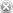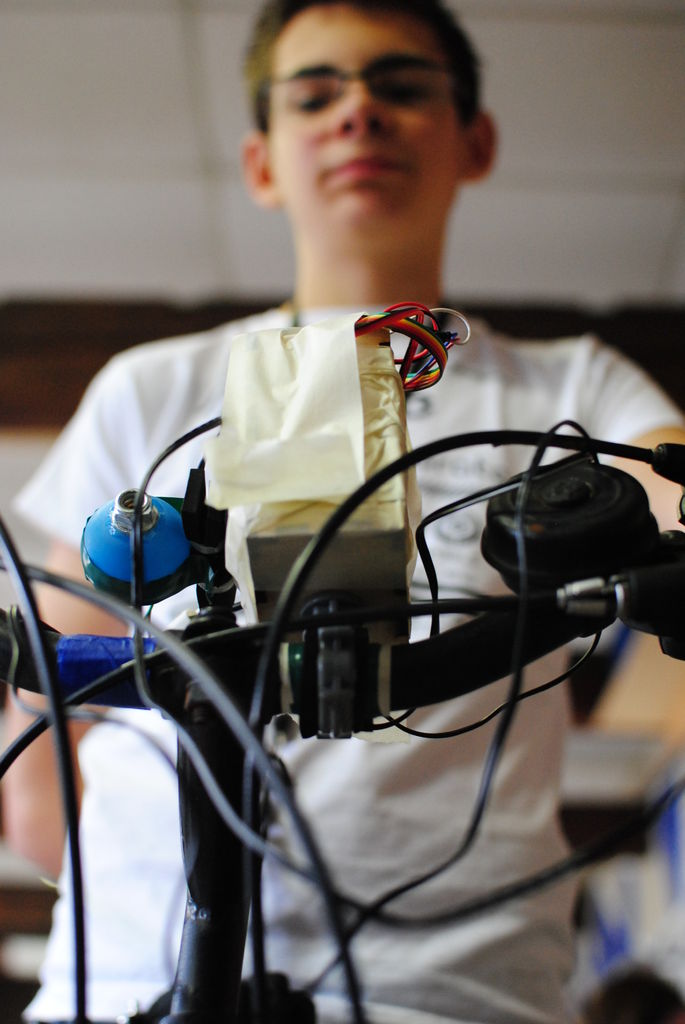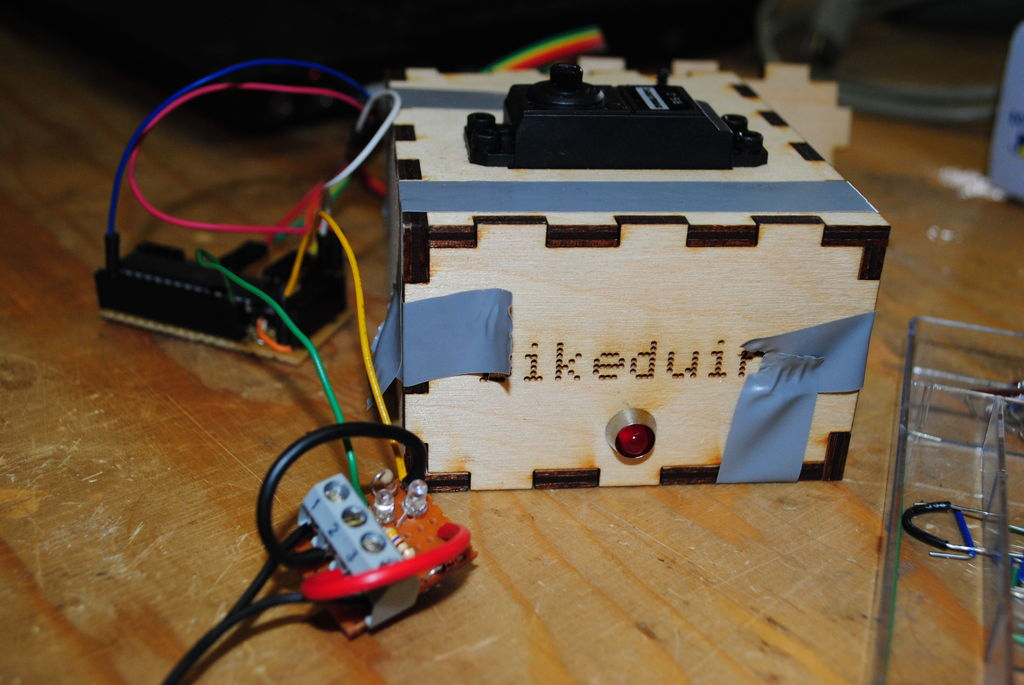# BIKEDUINO – Stopping Point Predictor for bike riders

The project consists in a Arduino that gets the speed of the bike from a dynamo and it controls a laser which points always to the point where the bike is gonna stop if it continues going at the same speed.

Used Hardware:

Arduino mini Atmega328
Laser
Servo
Dynamo
Battery pack 4 x 1,5 VRemove these ads by Signing Up

## Step 1: 1 Step: Build up an Mini Arduino Board

Build up an Mini Arduino Board or if you use it an Arduino Uno you can ignore this step.

http://http:/arduino/.cc/en/Main/StandaloneAssembly## Step 2: Step 2: Wire the dynamo in order to read the pulses it gives to the Arduino.

Make a single wave rectificator for the dinamo and make it give positive pulses out. Then conect these pulses to an analog entry.
For assuring that the dynamo is giving pulses correctly use the osciloscope.

## Step 3: Step 2: Process the reading from the Dynamo and get the SPEED , ACCELERATION and DISTANCE

Get the data on the Arduino, and process de pulses in order to get the Speed, Aceleration, and distance depending on the pulses.

You need to acurate very precisely in counting the pulses and then calbulate the SPEED, ACCELERATION and DISTANCE.

Here you get the code.

// Bikeduino version 1
// Lets your bicycle point a laser at the spot where it will stand still if the deceleration remains constant.

#include <Servo.h>

Servo servo;

// analog pin
#define DYNAMO_PIN 5
// pwm digital pin
#define SERVO_PIN 10
// digital pin
#define LASER_PIN 11

READ  [Team PGH] – Prototype I Final Report: [Motion RC Car] using arduino

// time sample averaging window
#define WINDOW_SIZE 32
// acceleration averaging window
#define AWINDOW_SIZE 8

// the angle at which servo is pointing horizontally
#define SERVO_STRAIGHT_ANGLE 140
// the amount of milimeters the bike moves in one pulse from the dynamo (measured)
#define mm_per_pulse 16
// the amount of milimeters the laser is above the ground (on the bicycle)
#define bike_height 1030

void setup() {
Serial.begin(19200);
Serial.println(“Bikeduino version 1.”);
servo.attach(SERVO_PIN);
servo.write(SERVO_STRAIGHT_ANGLE);
pinMode(LASER_PIN, OUTPUT);
}int q[WINDOW_SIZE];
int q_p = 0;

void queue(int x) {
if (++q_p >= WINDOW_SIZE)
q_p = 0;
q[q_p] = x;
}

int average_pulsetime() {
int i;
long r=0;
for (i=0; i<WINDOW_SIZE; i++) {
r += q[i];
}
return (int) (r/WINDOW_SIZE);
}

int aq[AWINDOW_SIZE];
int aq_p = 0;

void aqueue(int x) {
if (++aq_p >= AWINDOW_SIZE)
aq_p = 0;
aq[aq_p] = x;
}

int average_acceleration() {
int i;
long r=0;
for (i=0; i<AWINDOW_SIZE; i++) {
r += aq[i];
}
return (int) (r/AWINDOW_SIZE);
}

void wait_for_pulse() {
/*
}
}
*/
}
}
}

void laser_off() {
digitalWrite(LASER_PIN, LOW);
}

void laser_on() {
digitalWrite(LASER_PIN, HIGH);
}

int v_prev;
unsigned long prev_t;

void loop() {
unsigned long t;
int dt;
wait_for_pulse();
t = millis();
dt = t – prev_t;
queue(dt);
prev_t = t;

dt = average_pulsetime();
int v = (1000*mm_per_pulse)/dt; // [v] = mm/s

int dv = v – v_prev;
v_prev = v;

int a = (int) ((1000*(long)dv) / dt); // acceleration in mm/s^2

aqueue(a);
//  Serial.println();
//  Serial.print(“a: “);
//  Serial.println(a);
a = average_acceleration();

//  Serial.print(“t: “);
//  Serial.println(t);
//  Serial.print(“dt now:”);
//  Serial.println(q[q_p]);
//  Serial.print(“dt av: “);
//  Serial.println(dt);
//  Serial.print(“v: “);
//  Serial.println(v);
//  Serial.print(“a: “);
//  Serial.println(a);
if (a == 0) {
// don’t change anything
}
else if (a < 0) {
// we are slowing down, point the laser
laser_on();
int d = (int) (-sq((long) v)/a/2); // distance (mm) until standstill assuming deceleration remains constant
double angle = (180.0/3.1416) * atan((double)bike_height / d); // angle between laser and forward horizontal
servo.write(SERVO_STRAIGHT_ANGLE-angle); // change the minus to a plus if your servo is turned around
//    Serial.println();
//    Serial.print(“d: “);

READ  Autonomous Control of RC Car Using Arduino

//    Serial.println(d);
//    Serial.print(“angle: “);
//    Serial.println(angle);

}
else {
// not slowing down
laser_off();
servo.write(SERVO_STRAIGHT_ANGLE); // point forward
}

}